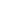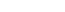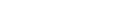# How to Use the Numpy Arctan Function

In this tutorial, I’ll show you how to use the Numpy arctan function to compute the trigonometric arctangent in Python.

I’ll explain the syntax of np.arctan, how the function works, and how to use it.

If you need something specific, just click on any of the following links.

Ok. Let’s get started.

## A Quick Introduction to Numpy Arctan

The Numpy arctan function computes the trigonometric arctangent in Python.Like many of the other trigonometric functions in Numpy – like Numpy cosine and Numpy sine – you can use Numpy arctan to operate on both single numbers and Numpy arrays.

I’ll show you how to do both, but first, we’ll look at the syntax.

## The syntax of np.arctan

The syntax for the Numpy arctan function is fairly simple:This, of course, assumes that you’ve imported Numpy with the alias np, which is the common convention.

#### Acceptable formats for the input

Let’s briefly discuss at the acceptable input types for the np.arctan function.

In the image of the syntax shown above, you can see that the input to the function is labeled “input“.

It’s important to mention that this input can take one of several different forms:

• a number (integer or float)
• a Numpy array (of integers or floats)
• a Python list of number, or list-like object

The np.arctan will operate on all of these inputs. Having said that, these different inputs will produce slightly different outputs

#### The output of np.arctan

The format of the output depends slightly on the input:

• if the input is a single number, then the output will be a single number (the arctangent of the input)
• if the input is a Numpy array, a Python list, or a list-like object, then the output will be a Numpy array of the arctangents

In addition to the input argument, the np.arctan function has some optional parameters:

• out
• where

These are somewhat rarely used, but so I won’t explain them here.

## Examples of how to use the Numpy arctangent function

Now that we’ve taken a look at the syntax for the Numpy arctangent function, let’s look at some examples.

Examples:

### Preliminary code: Import Numpy and Set Up Plotly

Before you run the examples, you’re going to need to run some preliminary code.

Specifically, you’ll need to import the packages we’re going to use.

You also may need to set up image rendering for Plotly (if you’re using an IDE).

#### Import Packages

First, you can import Numpy and Plotly with this code.

import numpy as np
import plotly.express as px


Obviously, we’ll use Numpy to run the np.arctan function.

But we’ll also use Plotly to plot the arctan function in example 4.

##### Set Up Image Rendering

Next, if you’re using an IDE like Spyder or PyCharm, you may need to set up your IDE so that it will render output images from Plotly.

By default, Plotly is set up to send output visualizations to your browser. That’s fine if you’re working in a notebook, but if you’re using an IDE, it will just cause an error.

So if you’re using an IDE, you’ll need to tell Plotly to render the output visualizations as svg images.

You can do that with the following code:

(Note: if you’re using Jupyter, you can skip this code!)

import plotly.io as pio
pio.renderers.default = 'svg'


Once you’re run the setup code, you should be ready to run the examples.

### EXAMPLE 1: Compute the arctangent of 0

Here, we’re going to compute the arctangent of 0.

np.arctan(0)


OUT:

0.0


#### Explanation

Obviously, this is very simple.

We’ve used np.arctan to compute the arctangent of 0, which is 0.0.

(Note that np.arctan outputs floating point numbers.)

### EXAMPLE 2: Compute the arctangent of 1,000,000

Now we’re going to compute the arctangent of 1,000,000.

np.arctan(1000000)


OUT:

1.5707953267948966


#### Explanation

Here, we’ve computed the arctan of 1,000,000.

Effectively though, what I’m doing is computing the arctangent of a relatively large number.

The output is 1.5707953267948966.

You might not recognize this, but it’s approximately.

This is important, because as,.

### EXAMPLE 3: Use the Numpy arctan function on a Numpy array of values

Next, we’re going to use the Numpy arctan function on a Numpy array.

Remember that np.arctan can operate on a single number (like in example 1 and example 2), but it can also operate on an array of numbers.

#### Create Numpy Array

First, let’s just create a Numpy array.

We’ll use Numpy linspace to create an array that contains 300 evenly spaced numbers from -10 to 10.

x_values = np.linspace(start = -10, stop = 10, num = 300)


#### Compute Arctangents using Numpy

Next, we’re going to compute the arctangent of every number in our array, x_values.

To do that, we provide x_values as the input to the function:

arctan_values = np.arctan(x_values)


Let’s take a look at the first few values of arctan_values, so we can see what’s inside:

arctan_values[1:10]


OUT:

array([-1.47046099, -1.46978535, -1.46910058, -1.4684065 , -1.46770291,
-1.46698962, -1.46626643, -1.46553313, -1.46478951])


#### Explanation

This is fairly straightforward.

Here, np.arctan has computed the arctangent of every element in x_values.

### EXAMPLE 4: Plot the arctangent function using Plotly

Finally, let’s plot the arctangent function.

To do this, we’ll use Plotly to plot the values in arctan_values, which we computed in example 3.

Specifically, we’ll use the Plotly line function, px.line, to plot the data:

px.line(x = x_values, y = arctan_values)


OUT:#### Explanation

Here, we’ve plotted the arctangent function with the Plotly line function.

We’ve plotted the values in x_values on the x-axis.

And we plotted the values in arctan_values, which we computed with np.arctan, on the y-axis.

Do you have other questions about how to use np.arctan?

In this tutorial, I’ve explained how to compute the trigonometric arctangent in Python using Numpy arctan.

This should help you with computing cosines and doing trigonometric operations, but if you really want to learn Numpy, there’s a lot more to learn.

If you’re serious about mastering Numpy, and serious about data science in Python, you should consider joining our premium course called Numpy Mastery.

Numpy Mastery will teach you everything you need to know about Numpy, including:

• How to create Numpy arrays
• How to reshape, split, and combine your Numpy arrays
• What the “Numpy random seed” function does
• How to use the Numpy random functions
• How to perform mathematical operations on Numpy arrays
• and more …

Moreover, this course will show you a practice system that will help you master the syntax within a few weeks. We’ll show you a practice system that will enable you to memorize all of the Numpy syntax you learn. If you have trouble remembering Numpy syntax, this is the course you’ve been looking for.

Find out more here:

Joshua Ebner

Joshua Ebner is the founder, CEO, and Chief Data Scientist of Sharp Sight. Prior to founding the company, Josh worked as a Data Scientist at Apple. He has a degree in Physics from Cornell University.